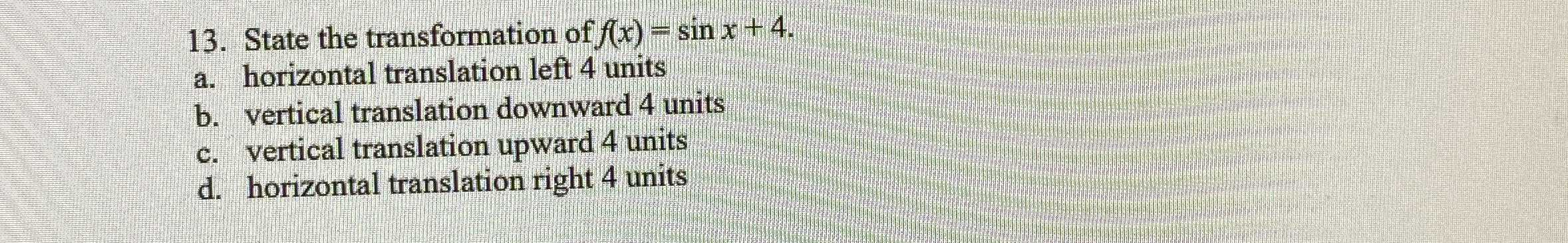### ¿Todavía tienes preguntas de matemáticas?

Pregunte a nuestros tutores expertos
Algebra
Pregunta13. State the transformation of $$f ( x ) = \sin x + 4 .$$

a. horizontal translation left $$4$$ units

b. vertical translation downward $$4$$ units

c. vertical translation upward $$4$$ units

d. horizontal translation right $$4$$ units